# Using an Arduino to monitor mains power usage

## Warning!

Parts of the circuits in this design are live. Mains electricity can be lethal. Unless you understand the risks, do not attempt to build or use this circuit. You have been warned.

## What it is for?

By monitoring your power usage you can get a good insight into the cost of running appliances. Without measurements you are left guessing how much power things use. I was surprised to find that my printer used 20W even in standby. Switching it off when not in use saves £20 a year.

By monitoring your power consumption in detail it is possible to detect when appliances switch on and off. Each appliance has a characteristic signature. It is possible to measure this, so you can tell not only how much power is used, but which appliances are on. This allows you to accurately cost each appliance. For example, my fridge has a characteristic switch-on profile. It draws around 1700W for 0.8s, a switch-on surge, then settles down to 80W. It will run for 07:30 every 36:00, so uses around 80 * (7.5/36) = 16.6W continuous. That is not bad. It should be possible for software to measure the efficiency of your fridge and calculate how much you can save by buying a more efficient one. You can multiply the continuous Wattage by 24 * 365 and divide by 1000 to calculate the annual kWh figure. By multiplying by your cost per kWh from your provider, you can calculate the total cost per year.

Monitoring can also be used for security and safety. If you are one of those people who always thinks they've left the cooker on when they leave the house, this system can tell you. If you are away, you could set an alarm if someone turns power on unexpectedly. You can see how many times you boil a kettle. You can even see if the gas boiler has fired as it has a pump and valves that can be detected, so you may be able to calculate your gas consumption too.

## Voltage monitoring

Warning! Just to remind you that this circuit contains lethal voltages. Do not attempt to make this circuit unless you understand what you are doing.

To measure power we need to measure the voltage and the current and multiply them together. The most popular way of monitoring the voltage is by using a transformer. This has a big advantage of electrically isolating the circuit from the mains. The main disadvantage is that a transformer is non-linear and will introduce distortion into the waveform. A waveform I measured from the output of a transformer is shown below. Pretty horrible.I decided to use a linear system to get a more accurate reading. First I looked at using opto-isolated linear amplifiers. These work by having a voltage-to-frequency converter on the mains side, driving an opto-isolator with the frequency pulses, then converting back to voltage again, eg, the HCPL-7520 Isolated Linear Sensing IC from Agilent. As I wanted to digitise the voltage, it seemed daft to convert the voltage to and from frequency as well. So, I decided to put an ADC on the mains side and transmit the digitised signal across an opto-isolator. The circuit I came up with is this :Be very very carefull about how the power supplies are connected. There are 2 sets of rails, 0V1, 5V1 for the 'hot' side and 0V2, 5V2 for the microcontroller side. They must not be connected together. I use an op-amp to generate a mid-rail voltage reference (2.5V). Because the 'hot' side of the circuit is floating, I can attach the voltage reference to the voltage divider on the mains input, and reference everything to this. The voltage divider was chosen to give near full scale out of the ADC when the maximum AC voltage is present. I'm in the UK so have 220V AC at 50Hz. You will need a different divider for 120V systems. The Schottky diodes are used to prevent input spikes from damaging the ADC. Power is provided by a small linear regulator and transformer.

An alternative to using opto-isolators that I considered was this iCoupler from analog devices. I might use these in a future design. It can also provide the DC power for the 'hot' side circuit which is nice.

The MCP3002 ADC has 10-bit resolution with a SPI interface. It is pretty fast, converting in 10..15µs, much faster than the Arduino's on-board ADC. It has 2 inputs. One is connected to the voltage divider, the other to the reference voltage. This allows easy measurement of the reference voltage, so the true AC voltage can be calculated.

I bought the ADCs from RS components. They turned up in a very large box. Why do companies do this? I placed a 50p coin next to it for scale.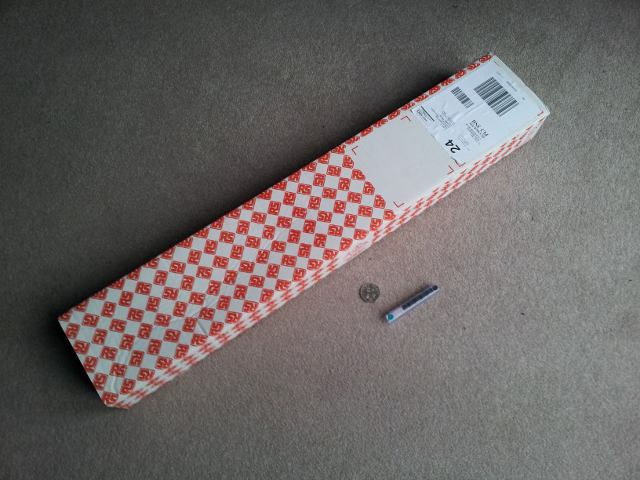Most optos aren't fast enough to transmit the SPI data, so I had to get high speed ones. I chose the VO2630. It is a dual channel opto with open-collector output and runs up to 10MHz. I needed 3 of these, 2 to send clock (SCK), data (MOSI) and chip select (CS) to the ADC, one to return the data. So that the SPI bus can still be used by other devices, I have a 1kΩ pull-up resistor attached to a control port on the MISO line. When the port is placed in tri-state, the MISO line is free for other devices to use.

It took a while to get the ADC working. It has a max clock speed of 200kHz, so I needed to divide the Arduino processor clock down. The microcontroller uses 8-bit SPI transfers, so you need to re-align the 10-bit ADC transfers by delaying the 'start conversion' bit by one. The ADC requires a low-impedance source of less than 1.5kΩ. Once I'd fixed this and put some 1kΩ pull-ups on the opto isolator, all worked fine. Reading the manual more carefully would have helped here. Here is a photo of the ADC and opto interface during development. No dangerous voltages yet.The prototype mains voltage interface board looks like this. I added links so that the dangerous parts of the circuit can be isolated for testing. The Arduino connects to the plug on the left. The 3 optos on the left divide the circuit between safe and dangerous.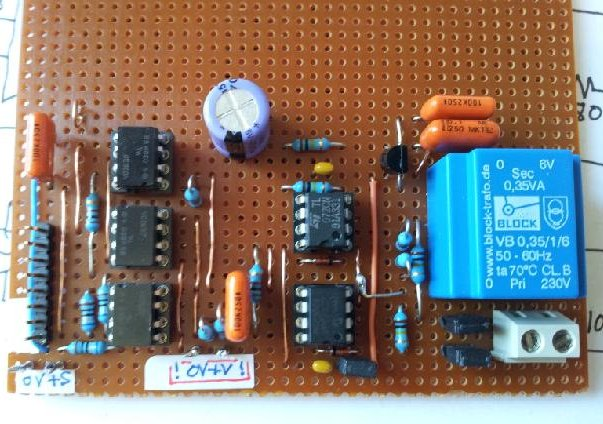reverse

I could now get the first results reading the mains voltage. I was expecting it to be more sinusoidal, but it has clipped peaks. This is probably because all the DC power supplies in the country pull current just at the peak, the cumulative effect being to reduce them. This graph shows that the peak is too near the maximum 512 ADC value for comfort. As a result I reduced the voltage on the divider by using a lower value R.## Current Monitoring

In a standard electricity meter the current would be measured using a shunt, producing a low voltage proportional to the current flowing. This would be the most accurate approach. Unfortunately I can't easily put the meter in series with the supply, so I use a current transformer instead. This seems to be standard for this sort of device.

The excellent Open Energy Monitor site has lots of useful information on CT designs.

The circuit is much simpler than voltage side. An op-amp provides a low impedance voltage reference. The current transformer drives a 33Ω burden resistor, feeding a programmable gain amplifier (PGA), in this case the MCP6S91. This provides 1 to 32 gain, controlled over a SPI interface. The PGA is needed to improve the dynamic range of the converter, so we can detect small currents as well as large ones. It effectively gives us an extra 5 bits resolution at the low end. A pair of Schottky diodes protect the amplifier from transients, the Arduino's ADC inputs are used to measure both the output of the PGA and the reference voltage.## Putting it together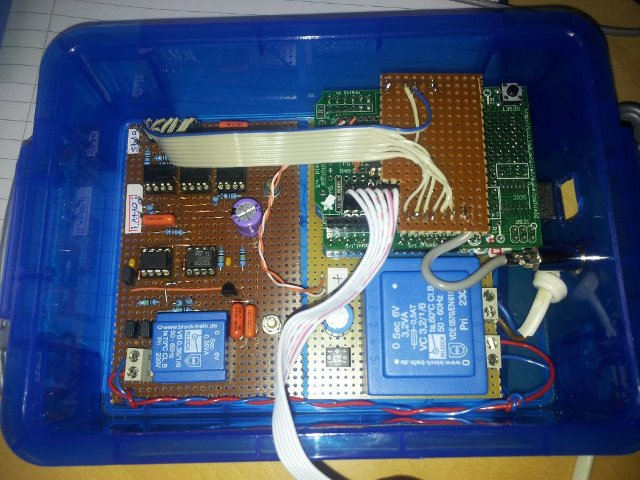To calculate the power in Watts you need to multiply voltage by current. The power needs to be summed over time, so you need to take a number of samples in each mains cycle and add them together. I decided to take 128 samples per cycle, at 50Hz, this is 128 * 50 = 6400Hz, or ~156µs per sample. I and V are 10-bits each. The I reading has an additional max *32 gain, giving 15-bits. The product of I & V is therefore 10 + 15 = 25 bits. By summing 128 of these products, we get a further 7 bits resolution, making a total of 32 bits. So the sum of products for one cycle will just fit in a 32-bit word.

I use a timer interrupt to drive the sample rate. The standard Arduino library ADC read function is blocking and takes over 110µs, which is most of the available 156µs sample period. So, the ADC read is split into 2 parts, start conversion, and read result. By reading the previous result then starting again in each timer interrupt, the conversion can take place in the background. This frees up the time required to get the voltage ADC reading and push the voltage and current data to a queue.

I read the voltage ADC in the timer interrupt, which is a bit naughty as it blocks. However it only takes around 15µs. An interrupt driven solution would require 2 interrupts, as the Arduino SPI transfers are only 8-bit, and the interrupt overhead would add to nearly the same time. I also need to make occasional PGA adjustments in the interrupt handler. This must be done prior to the current monitor's start conversion signal, to ensure that the ADC input is fixed during the long conversion period.

The main loop polls the queue for data, pulling the data, multiplying and summing. I avoid floating point calculation as much as possible as it is really slow. It can be reduced to a single floating point multiply per mains cycle. I found some benchmarks on Arduino maths here. I don't use any calibration technique, but rely on calculating the results using the known component values, shown here in Python. I have ignored the component tolerances. It is more complicated if you use a transformer for the voltage reading, as they have a variable no-load gain.

```# Current transformer : 2000 turns, 33ohm burden.
# 2.5V max, i_line = 2000 * (2.5 / 33)
# Voltage : 200k / 1270R resistor chain.
# i_max = 2.5/1270, v_max = i_max * (200k + 1270)

v_max = 2.5
i_turns = 2000
r_burden = 33
r_1 = 200000
r_2 = 1270
c_max = 512
v_line = (v_max / r_2) * (r_1 + r_2)
i_line = i_turns * (v_max / r_burden)

# 10-bit adc, gives +- 512 counts for 2.5V signal excursion
factor = ((v_line / c_max) * (i_line / c_max))
```

The power in Watts can then be calculated by using a single floating point multiply. I do not currently calculate power factor etc. just the instantaneous power, summed over the cycle.

The Arduino source code is here

The power reading is output on each cycle to the serial interface. I read this with a laptop (at the moment) which logs the data, pushes it to cosm and provides graphs. The graph below shows a combination of solar PV generation and kettle etc. on a very dull day. The solar input massively complicates the waveform and my inverter seems to be very noisy. It looks like I'm going to have to add a second CT so I can measure the solar data separately.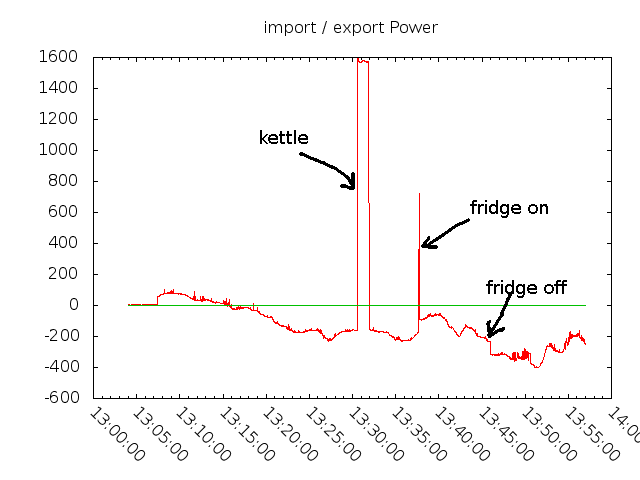Here is the fridge switch on in detail, several instances normalised and overlaid to show how repeatable it is. The x-scale is in 1/50 seconds, y in Watts. I wonder if it is possible to detect fridge compressors wearing out, before they fail?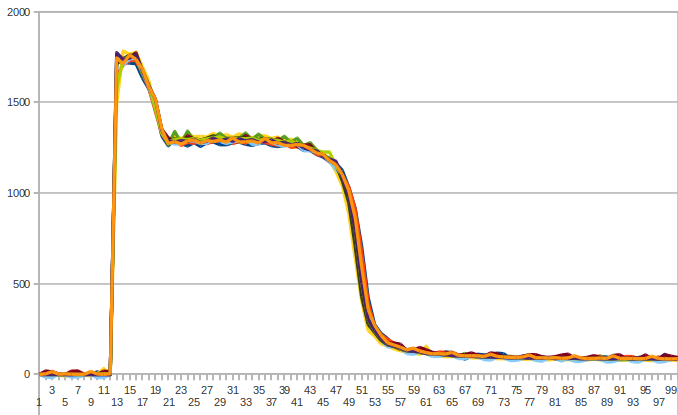And here is a whole day. A dull day, 28-Jun-2012 (a bad Summer) particularly at lunchtime when I obviously cooked something when the solar panels were struggling. You can see the periodic fridge activity. And then a burst of activity around midnight. Not cooking this time, but ironing. Not me. But another good reason not to iron. The total import / export data shows that we are exporting most of our generated energy. The tariff works by assuming that you use half the energy you generate, so you only get paid for half. It might be an advantage to get a proper export meter fitted and to change the agreement so we get paid more. The generation meter read 9.27 for this day, so at least we are using some of our own energy.## Postscript

Comparing my solar export data with the output of this circuit, I see that it is out by several percent. It does need calibration. I will add this at some stage.

Since this article was posted on Hack-a-day I had the suggestion that it would be better to use a dedicated chip to carry out the measurement. The one suggested, the Cirrus CS5463, does look good. It will produce a cheaper and more accurate result than my circuit. Perhaps combined with the iCoupler it would make an excellent design. It is limited in only providing a single channel, but that is all I'm measuring at present.

I've also come across a new chip, the SiliconLabs Si890x Isolated AC Mains Monitor, which has a 3 input analog MUX, a PGA, 10-bit ADC and opto-isolated SPI interface all on a single device. This makes a very simple low cost system that would be better than my design. It would work with either a current shunt or CT interface.

My design was partly educational : I learnt a lot going through the design process. I was also constrained by using through-hole parts, as I have no surface mount facilities. If I was making a PCB I would probably use different parts. The CS5463 would also make an excellent device for monitoring a single appliance. Combined with an improved version of my triac project, it would make a great smart power controller.